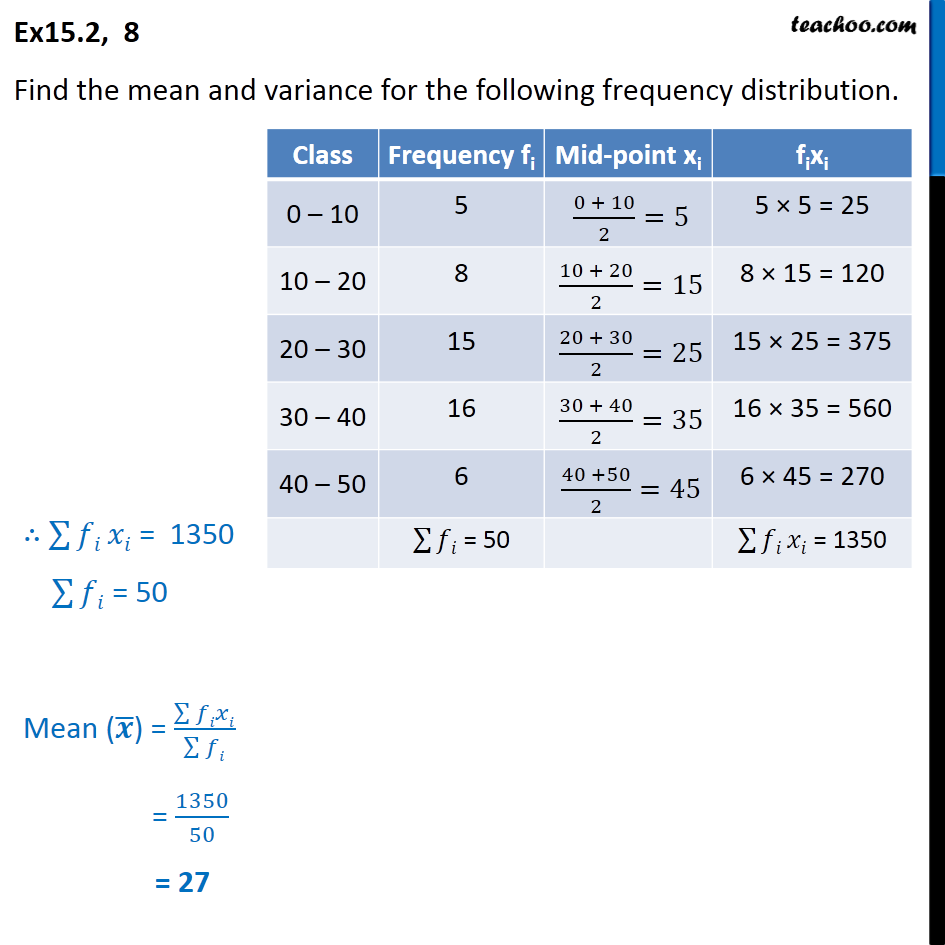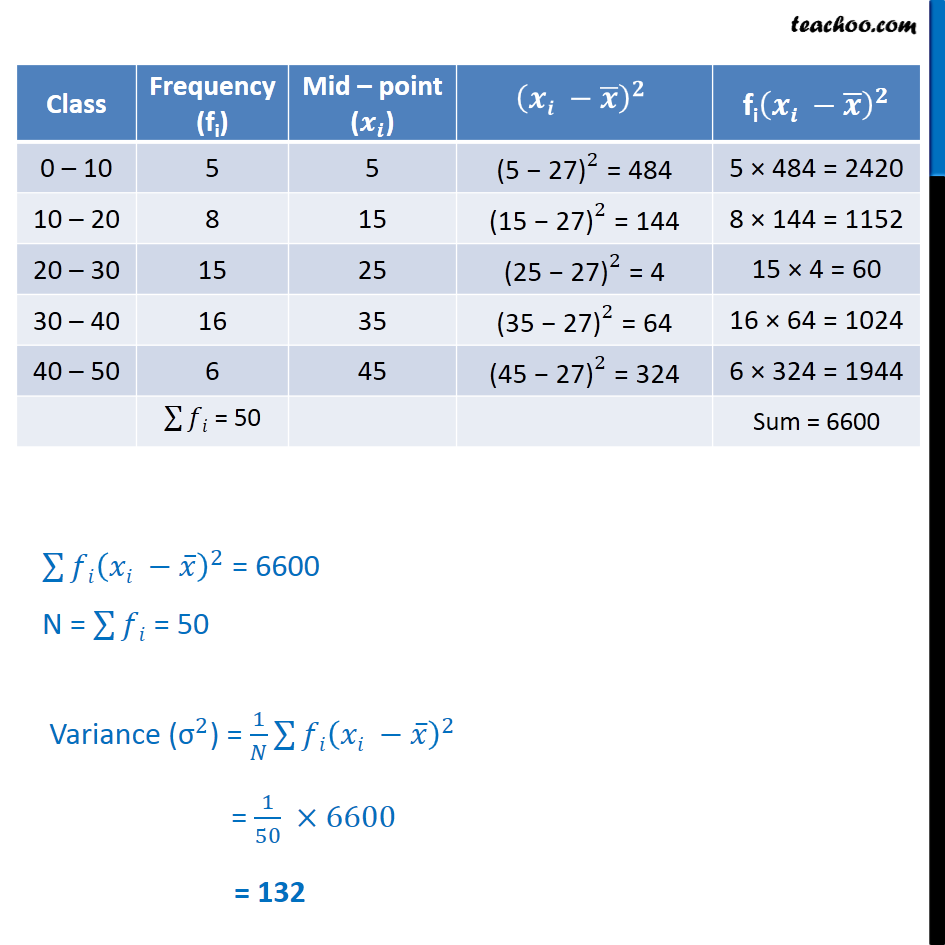Ex 13.2

Chapter 13 Class 11 Statistics
Serial order wiseLearn in your speed, with individual attention - Teachoo Maths 1-on-1 Class

### Transcript

Ex 13.2, 8 Find the mean and variance for the following frequency distribution. ( )^2 = 6600 N = = 50 Variance (" " ^2) = 1/ ( )^2 = 1/50 6600 = 132